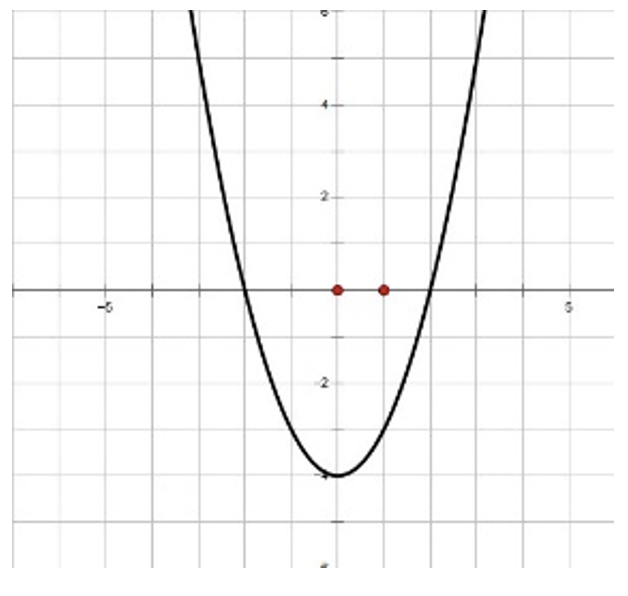# How do I use the Quadratic Formula?

What does the Quadratic Formula help us find? What is it exactly? To put it simply, the quadratic formula is an algebraic formula that allows you to find the x-intercepts or roots of a quadratic equation.## What does the Quadratic Formula help us find?

The quadratic formula is a formula that allows you to find the x – intercepts or roots of a quadratic equation.

A quadratic equation can have three different root(s) outcomes.

## How do I use the Quadratic Formula?

Now that you are familiar with the types of roots a quadratic equation can produce, let us look at the quadratic formula.

To effectively use the Quadratic Formula, we must know the standard form of a quadratic. This will be used to help you identify the values of a, b, and c.

### Quadratic Formula Problem Example 1:

Use the Quadratic Formula to solve,

#### Step 2: Identify your a,b, and c from your equation by looking at the general standard form equation.

a = 1, b = 6, and c = -16

#### Step 4: Simplify - Solve the Quadratic Formula!

So, the roots are at x = 2 and x = -8. There are two rational roots because the roots are rational numbers.

### Quadratic Formula Problem Example 2:

Use the Quadratic Formula to solve,

#### Step 2: Identify your a, b, and c from your equation by looking at the general standard form equation.

a = 1, b = -6, and c = 10

#### Step 4: Simplify - Solve using the Quadratic Formula!

So, the roots are at x= 3 + i and x = 3 - i, which are both complex roots because you can’t take the square root of a negative number. Thus, in the equation, the parabola does not hit the x-axis.

#### MORE MATH PROBLEMS# Need more help? Get Algebra or Geometry Tutoring

Remind Math Tutoring provides 1 on 1 online algebra tutoring. Your experienced tutor can help you work through difficult problems and help you master all the algebra concepts. How do I use the Quadratic Formula? No problem. The first session is free, and it's \$40 per session after that. If you need some Algebra help, get an Algebra math tutor to help.